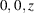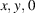International Tables for Crystallography (2006). Vol. A, ch. 4.1, pp. 56-60https://doi.org/10.1107/97809553602060000507

## Contents

• 4.1. Introduction to the synoptic tables  (pp. 56-60)
• 4.1.1. Introduction  (p. 56) | html | pdf |
• 4.1.2. Additional symmetry elements  (pp. 56-60) | html | pdf |
• 4.1.2.1. Integral translations  (pp. 56-57) | html | pdf |
• 4.1.2.2. Centring translations  (pp. 57-59) | html | pdf |
• 4.1.2.3. The priority rule  (pp. 59-60) | html | pdf |
• References | html | pdf |
• Tables
• Table 4.1.2.1. Location of additional symmetry element, if the translation vector t is perpendicular to the symmetry axis alongor to the symmetry plane in(p. 57) | html | pdf |
• Table 4.1.2.2. Additional symmetry elements and their locations, if the translation vector t is inclined to the symmetry axis or symmetry plane  (p. 58) | html | pdf |
• Table 4.1.2.3. Additional symmetry elements due to a centring vector t and their locations  (p. 59) | html | pdf |